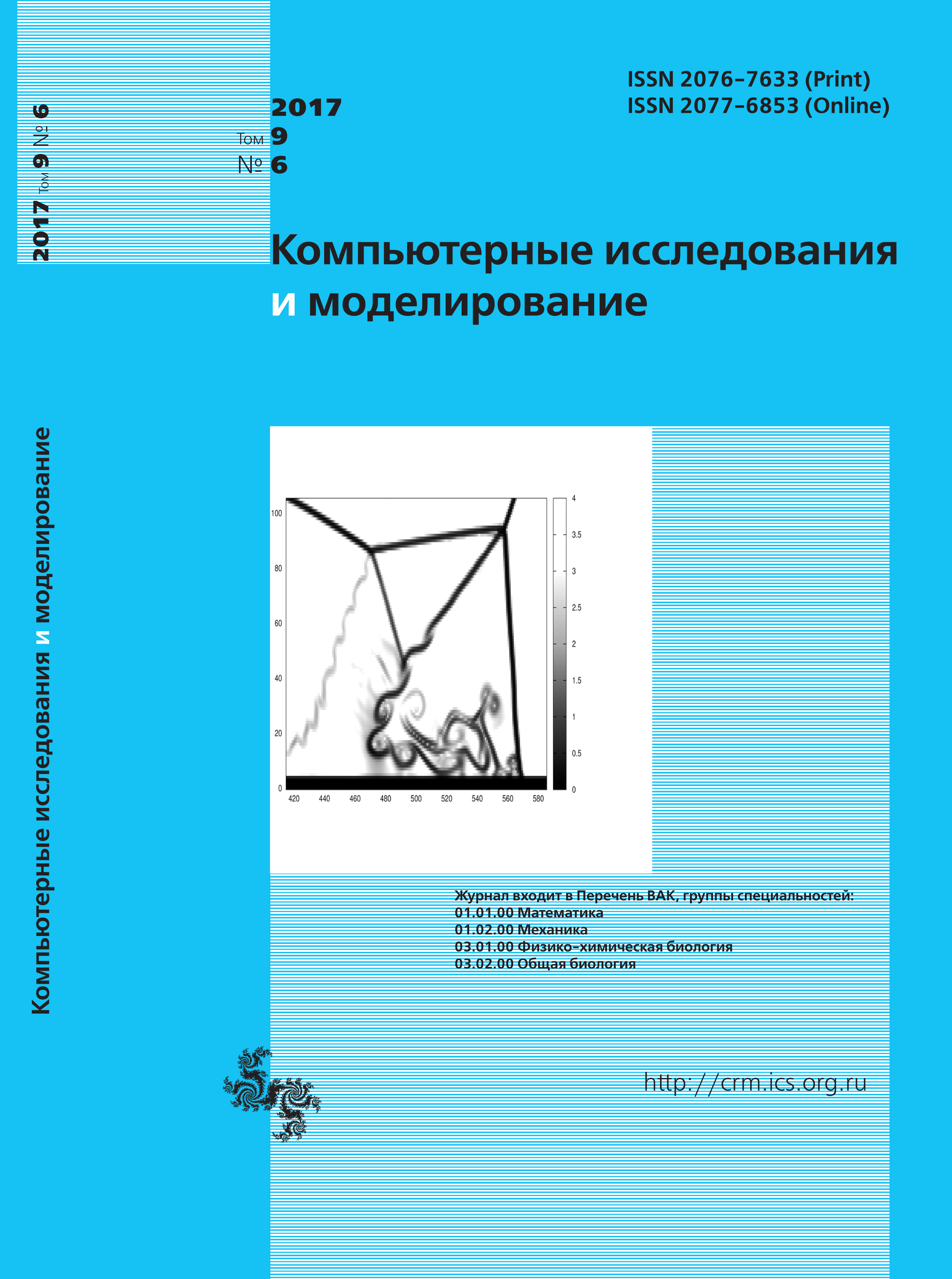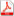Issue 6, 2017 Vol. 9

# All issues

On the convergence of the implicit iterative line-by-line recurrence method for solving difference elliptical equationspdf (414K)  / List of references

In the article a theory of the implicit iterative line-by-line recurrence method for solving the systems of finite-difference equations which arise as a result of approximation of the two-dimensional elliptic differential equations on a regular grid is stated. On the one hand, the high effectiveness of the method has confirmed in practice. Some complex test problems, as well as several problems of fluid flow and heat transfer of a viscous incompressible liquid, have solved with its use. On the other hand, the theoretical provisions that explain the high convergence rate of the method and its stability are not yet presented in the literature. This fact is the reason for the present investigation. In the paper, the procedure of equivalent and approximate transformations of the initial system of linear algebraic equations (SLAE) is described in detail. The transformations are presented in a matrix-vector form, as well as in the form of the computational formulas of the method. The key points of the transformations are illustrated by schemes of changing of the difference stencils that correspond to the transformed equations. The canonical form of the method is the goal of the transformation procedure. The correctness of the method follows from the canonical form in the case of the solution convergence. The estimation of norms of the matrix operators is carried out on the basis of analysis of structures and element sets of the corresponding matrices. As a result, the convergence of the method is proved for arbitrary initial vectors of the solution of the problem.

The norm of the transition matrix operator is estimated in the special case of weak restrictions on a desired solution. It is shown, that the value of this norm decreases proportionally to the second power (or third degree, it depends on the version of the method) of the grid step of the problem solution area in the case of transition matrix order increases. The necessary condition of the method stability is obtained by means of simple estimates of the vector of an approximate solution. Also, the estimate in order of magnitude of the optimum iterative compensation parameter is given. Theoretical conclusions are illustrated by using the solutions of the test problems. It is shown, that the number of the iterations required to achieve a given accuracy of the solution decreases if a grid size of the solution area increases. It is also demonstrated that if the weak restrictions on solution are violated in the choice of the initial approximation of the solution, then the rate of convergence of the method decreases essentially in full accordance with the deduced theoretical results.

Keywords: system of linear algebraic equations, iterative method, convergence of method
Citation in English: Fomin A.A., Fomina L.N. On the convergence of the implicit iterative line-by-line recurrence method for solving difference elliptical equations // Computer Research and Modeling, 2017, vol. 9, no. 6, pp. 857-880
Citation in English: Fomin A.A., Fomina L.N. On the convergence of the implicit iterative line-by-line recurrence method for solving difference elliptical equations // Computer Research and Modeling, 2017, vol. 9, no. 6, pp. 857-880
DOI: 10.20537/2076-7633-2017-9-6-857-880
Views (last year): 15. Citations: 1 (RSCI).

Full-text version of the journal is also available on the web site of the scientific electronic library eLIBRARY.RU

The journal is included in the Russian Science Citation Index

The journal is included in the List of Russian peer-reviewed journals publishing the main research results of PhD and doctoral dissertations.

International Interdisciplinary Conference "Mathematics. Computing. Education"

The journal is included in the RSCI

Indexed in Scopus